Question

Linear Algebra

Recall that Z denotes the ring of integers, and Q is the rationals. We let F[x]denote the polynomial ring in the variable.in F[x].over a field F, and we let f(x) = x²+1

\text { (1) Show that } f(x) \text { is irreducible when } F=Z /(7) \text {. }

Fx]/(f(x)) with F = Z/(3), and let a e E be a root of f(x).(2) Let E =Express g(1+a) when g(x) = 2x²+x²+1 in the basis {1, a} of the F-vector space E.

\text { (3) Assume that the integer } p \text { is a prime of the form } p=a^{2}+b^{2} \text {. Let } F^{\prime}=Z /(p) \text {. }

\text { Is the complex number } \frac{1}{4}(2+i \sqrt{3}) \text { algebraic (over the rationals)? }

\text { Is } x^{5}+5 x+5 \text { irreducible in } \mathrm{Q}[x] ?

\text { Factor } x^{4}+x^{2}+1 \text { into irreducible factors in } Q[x] \text {. }

') Determine the degree of the extension Q C E when E is the splitting field of the rational polynomial x4 + x² + 1

Show that a finite field F can not be algebraically closed.Verified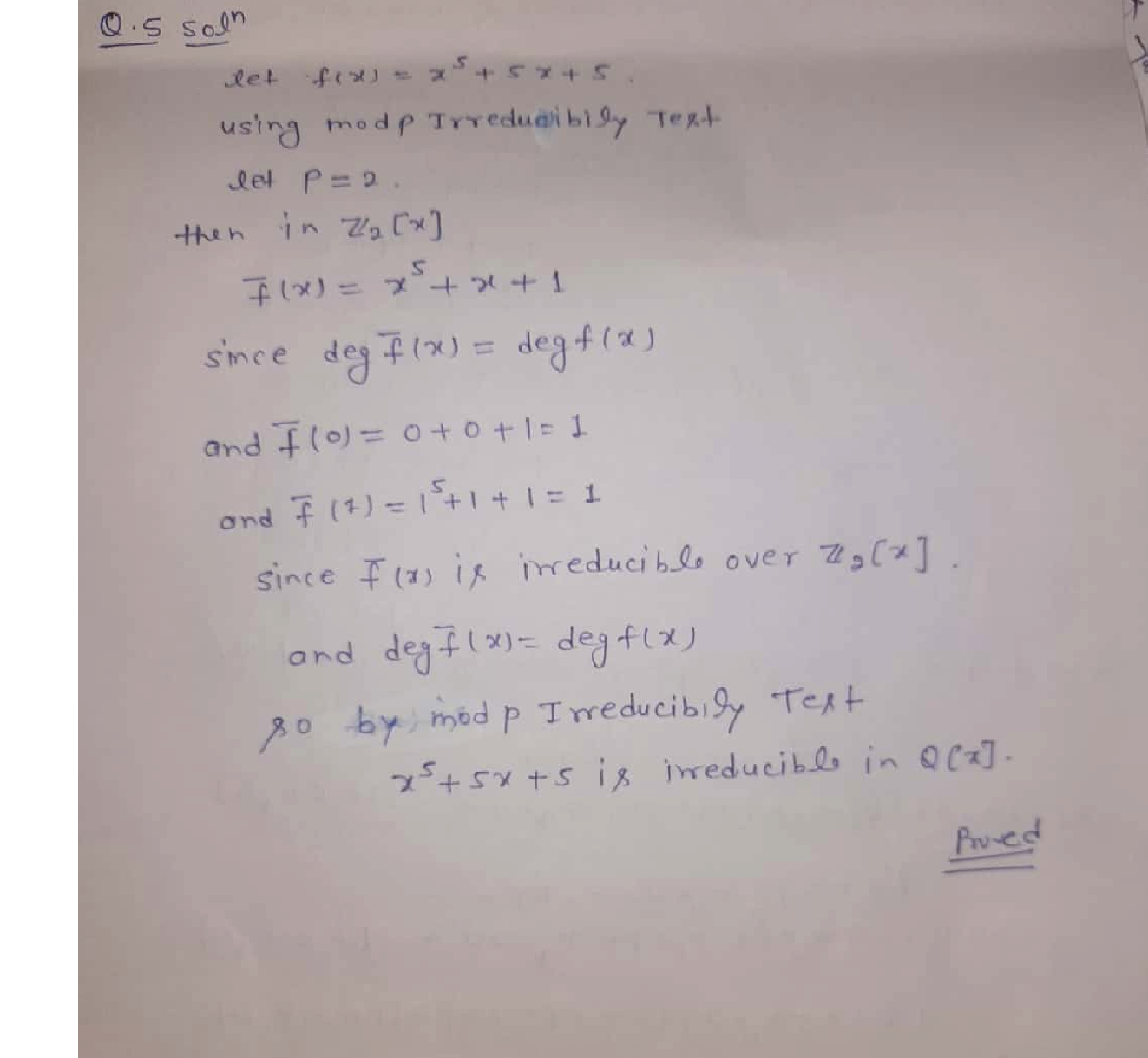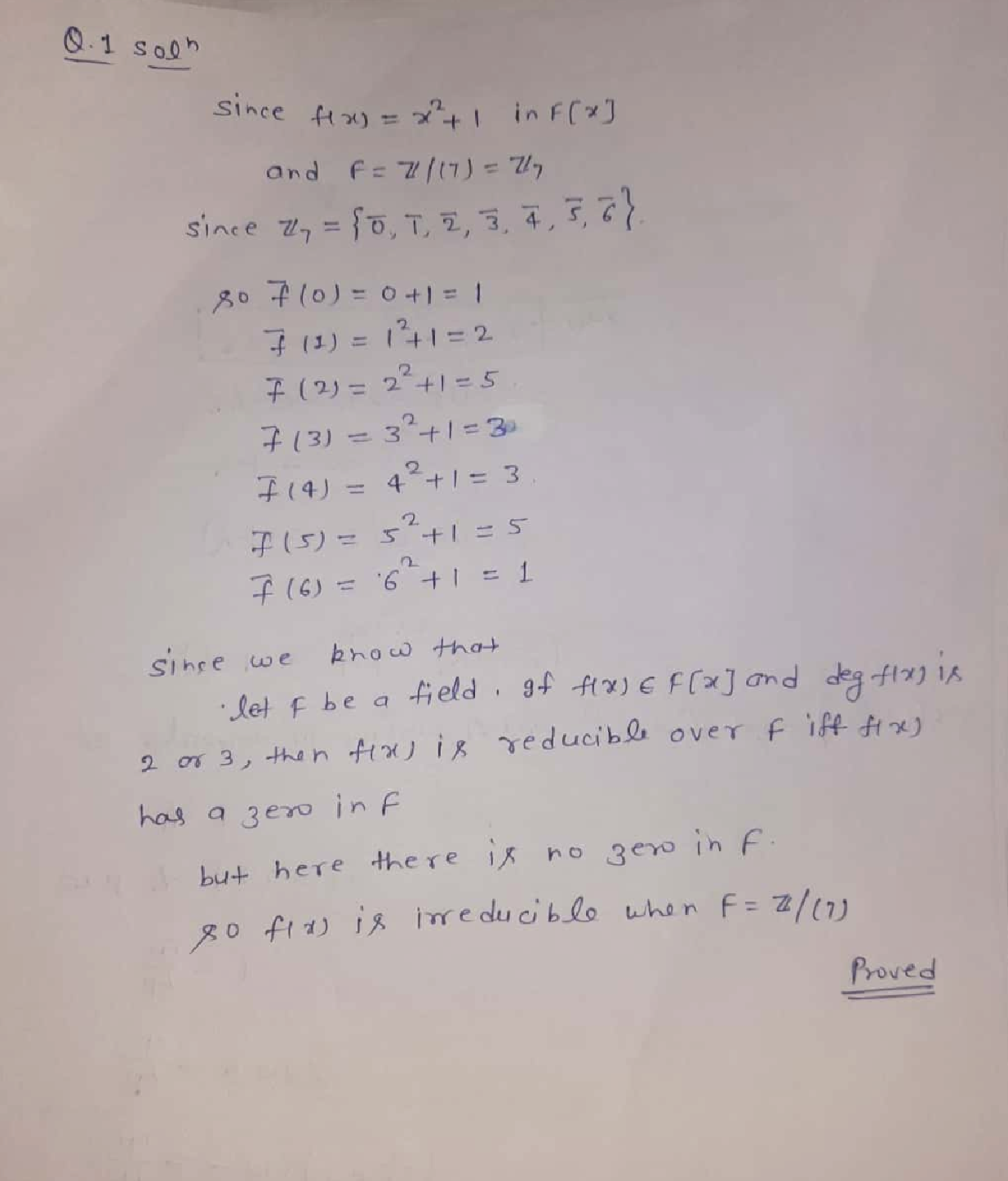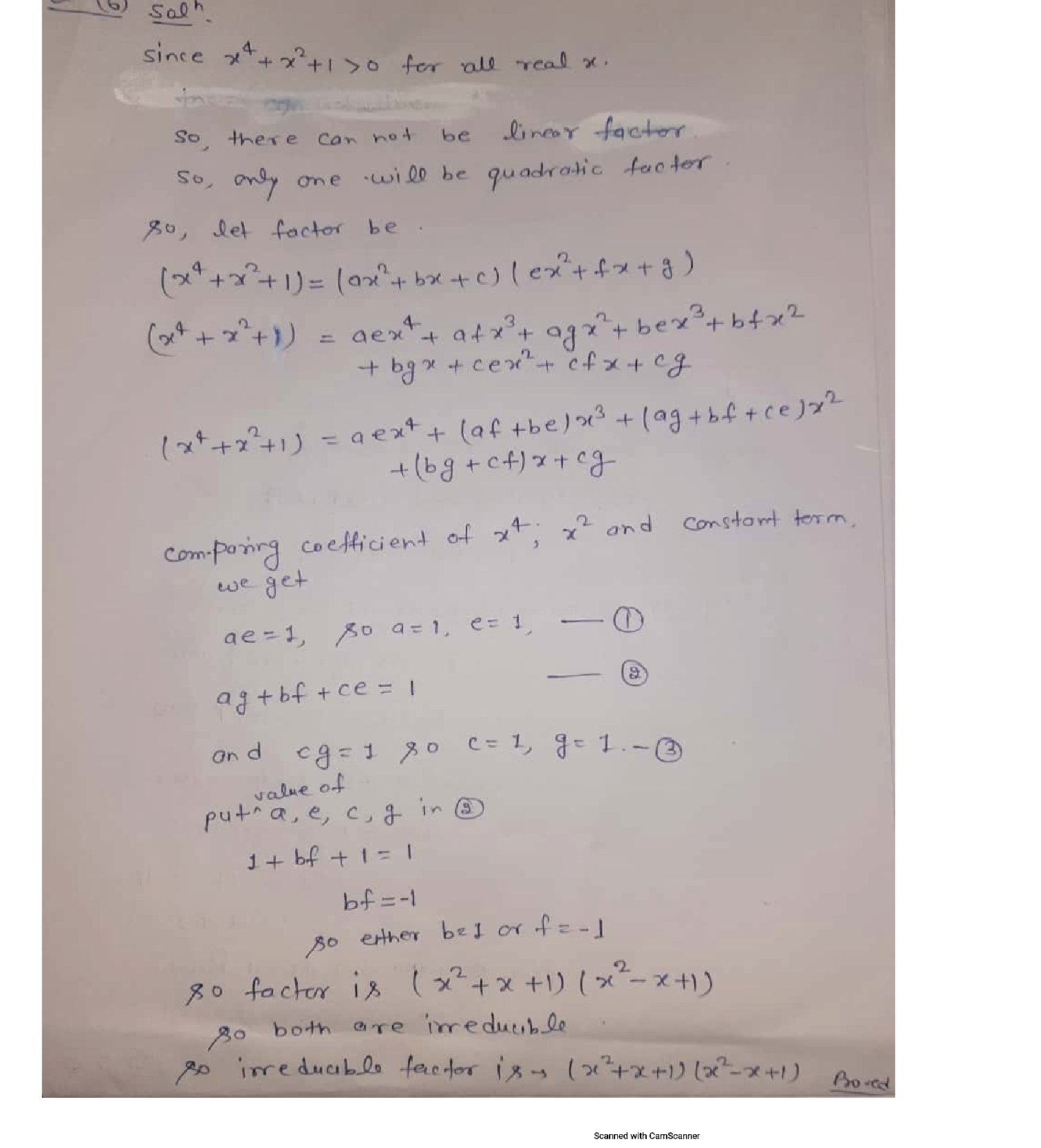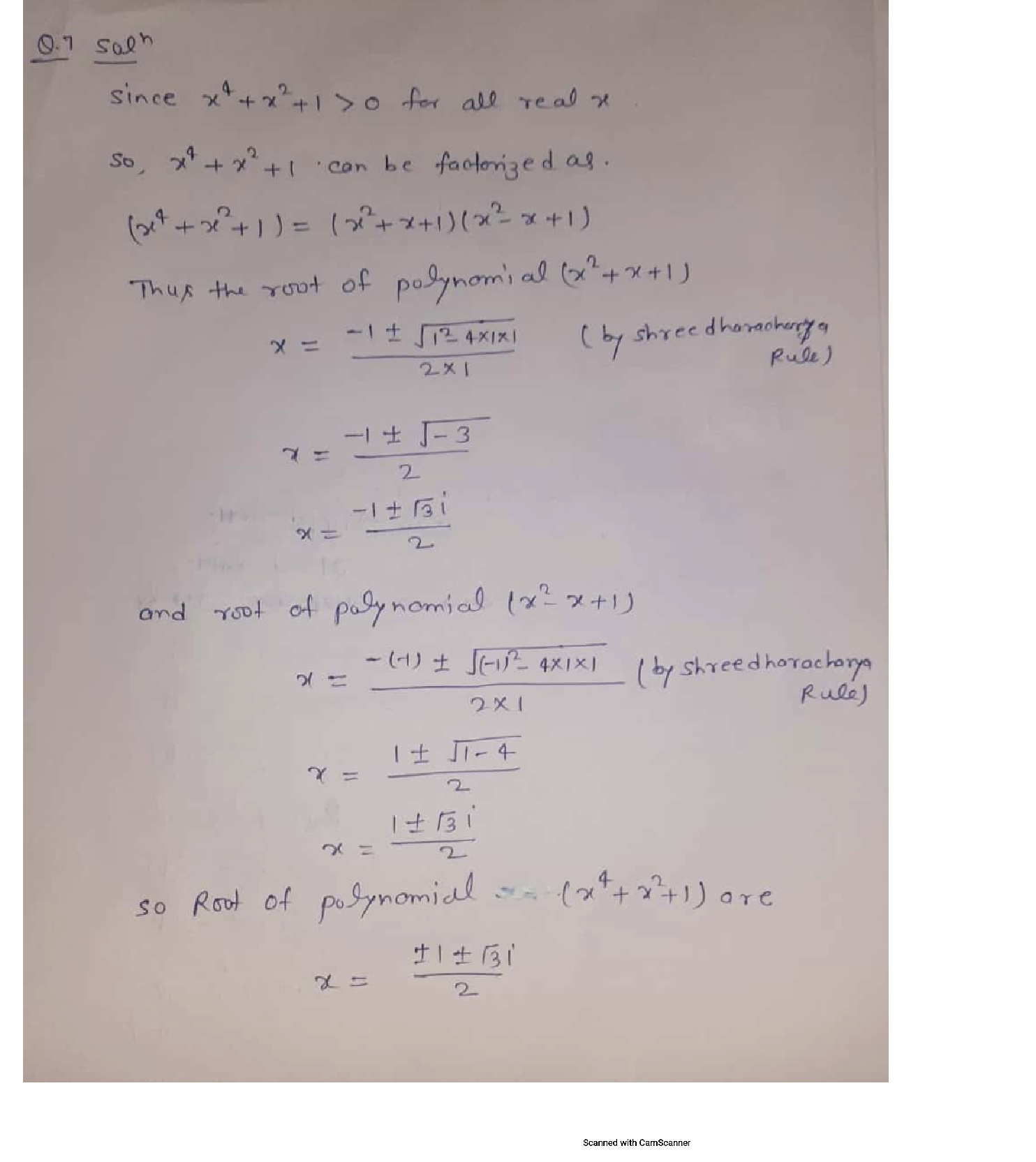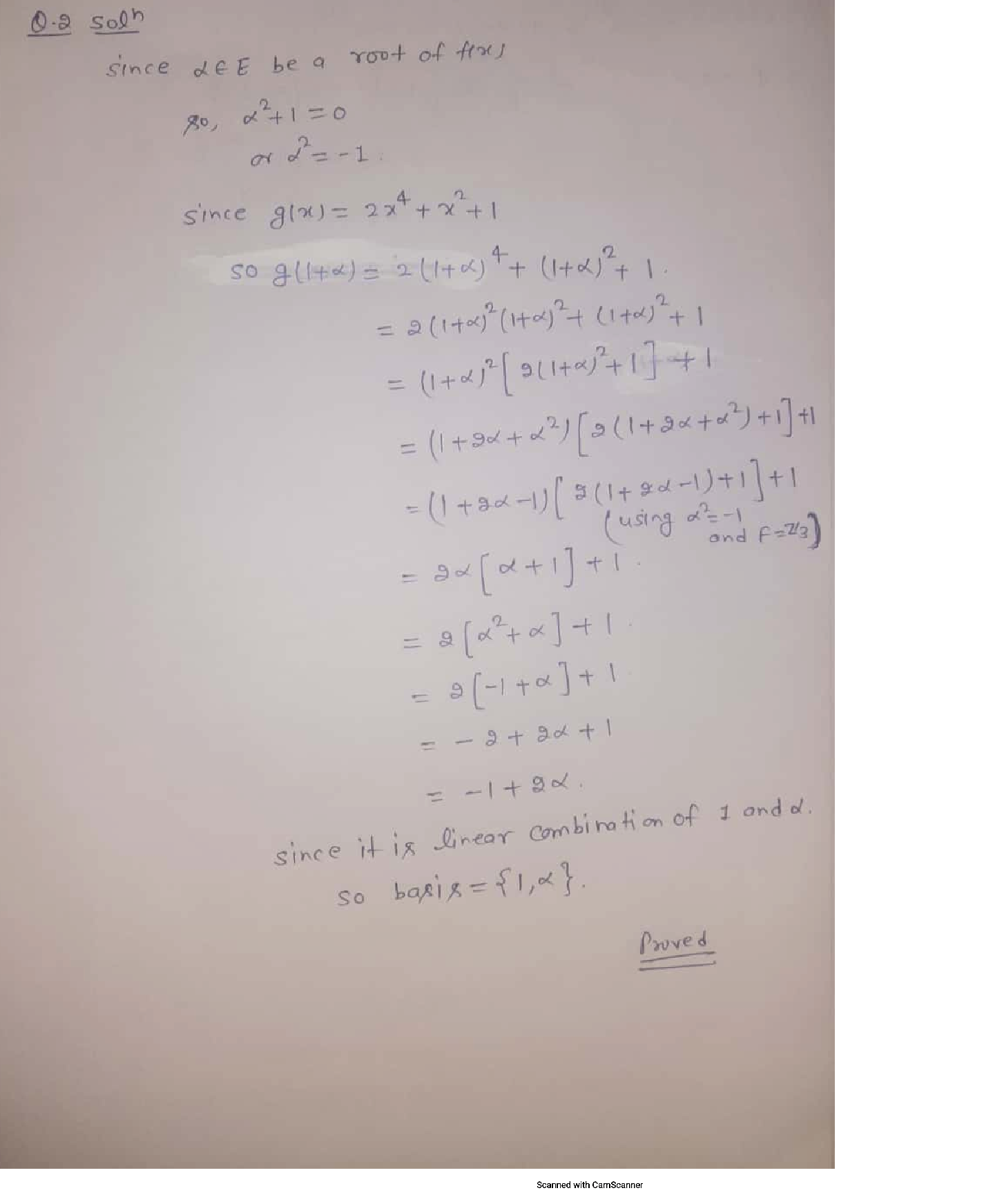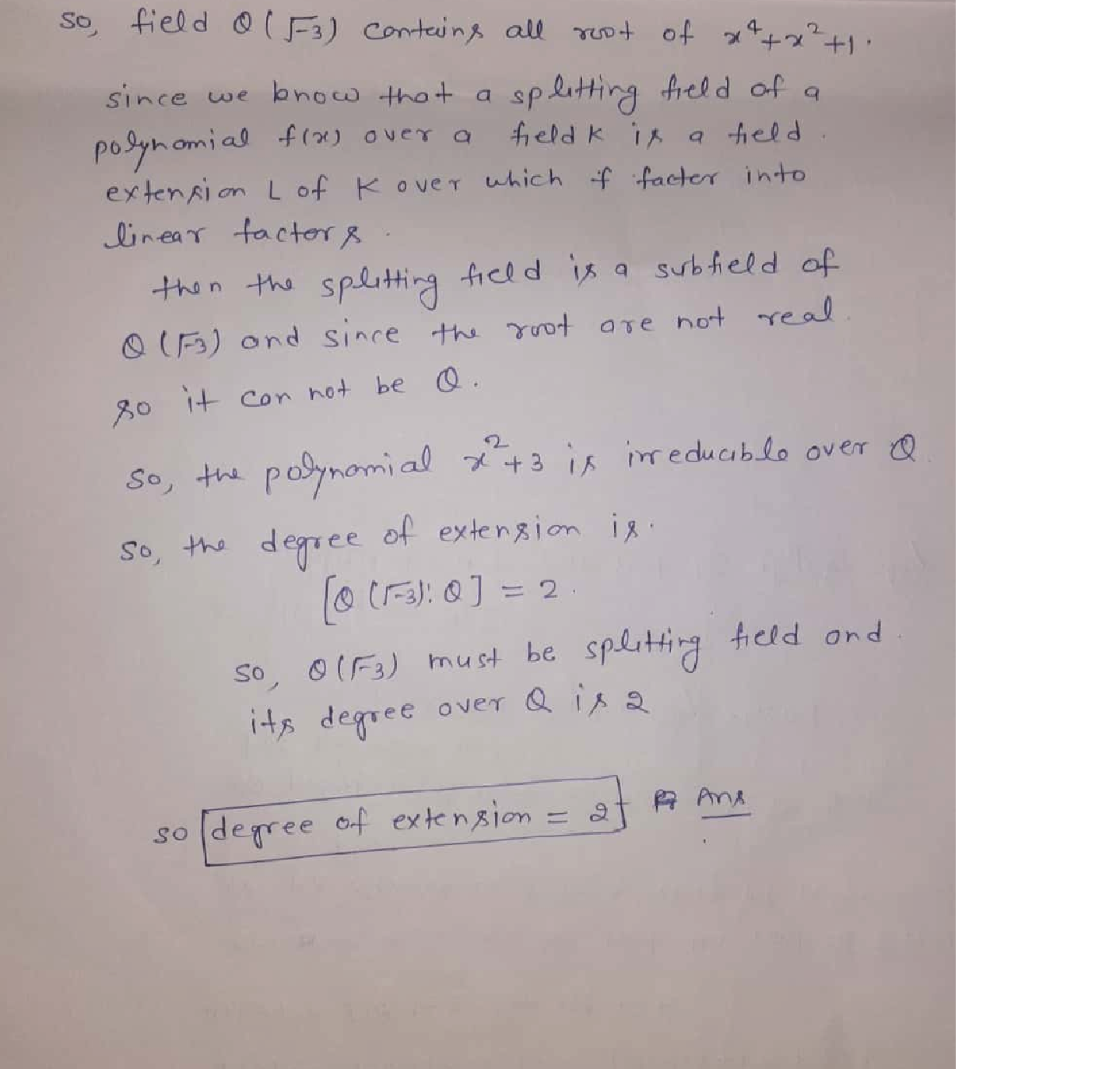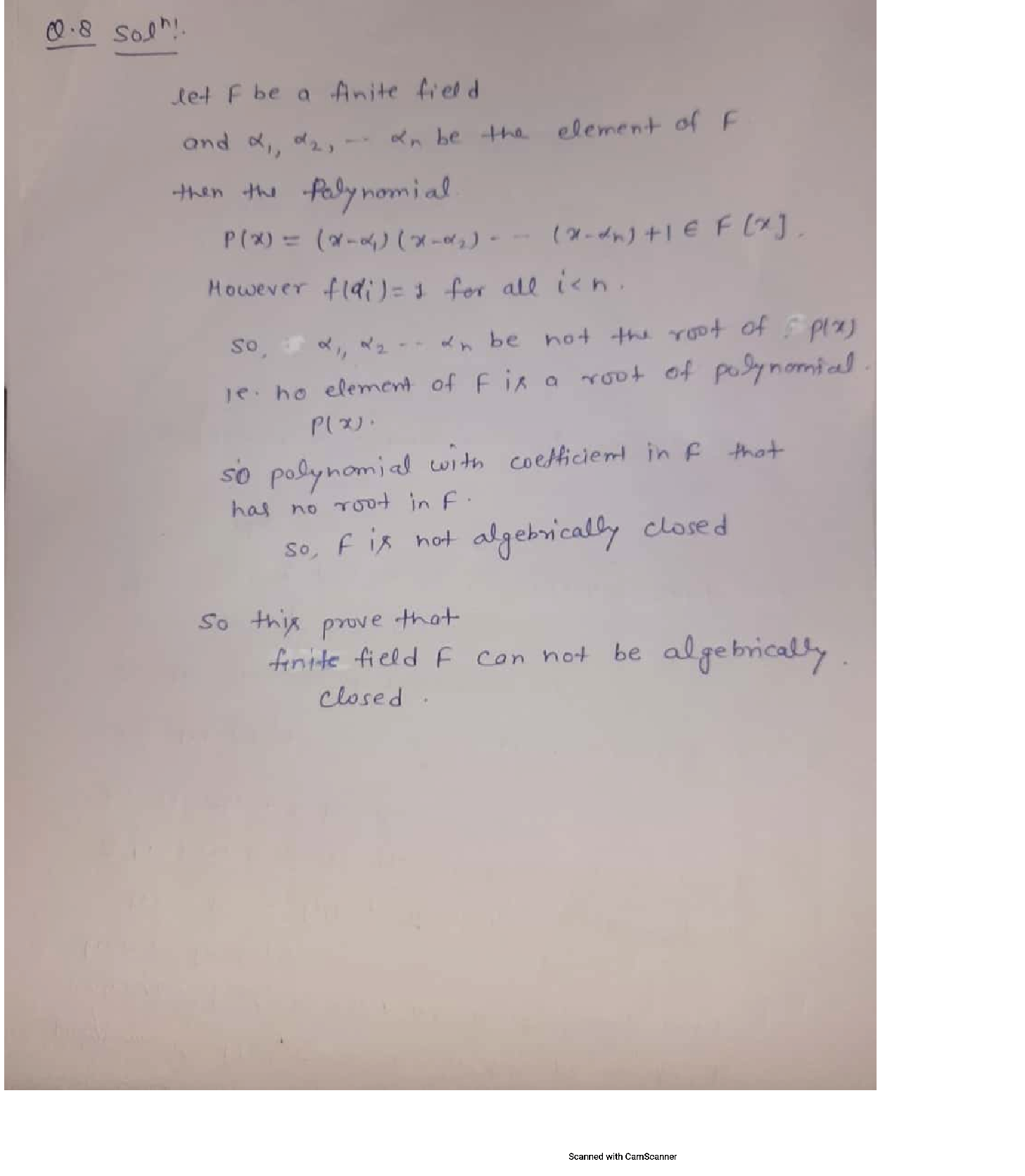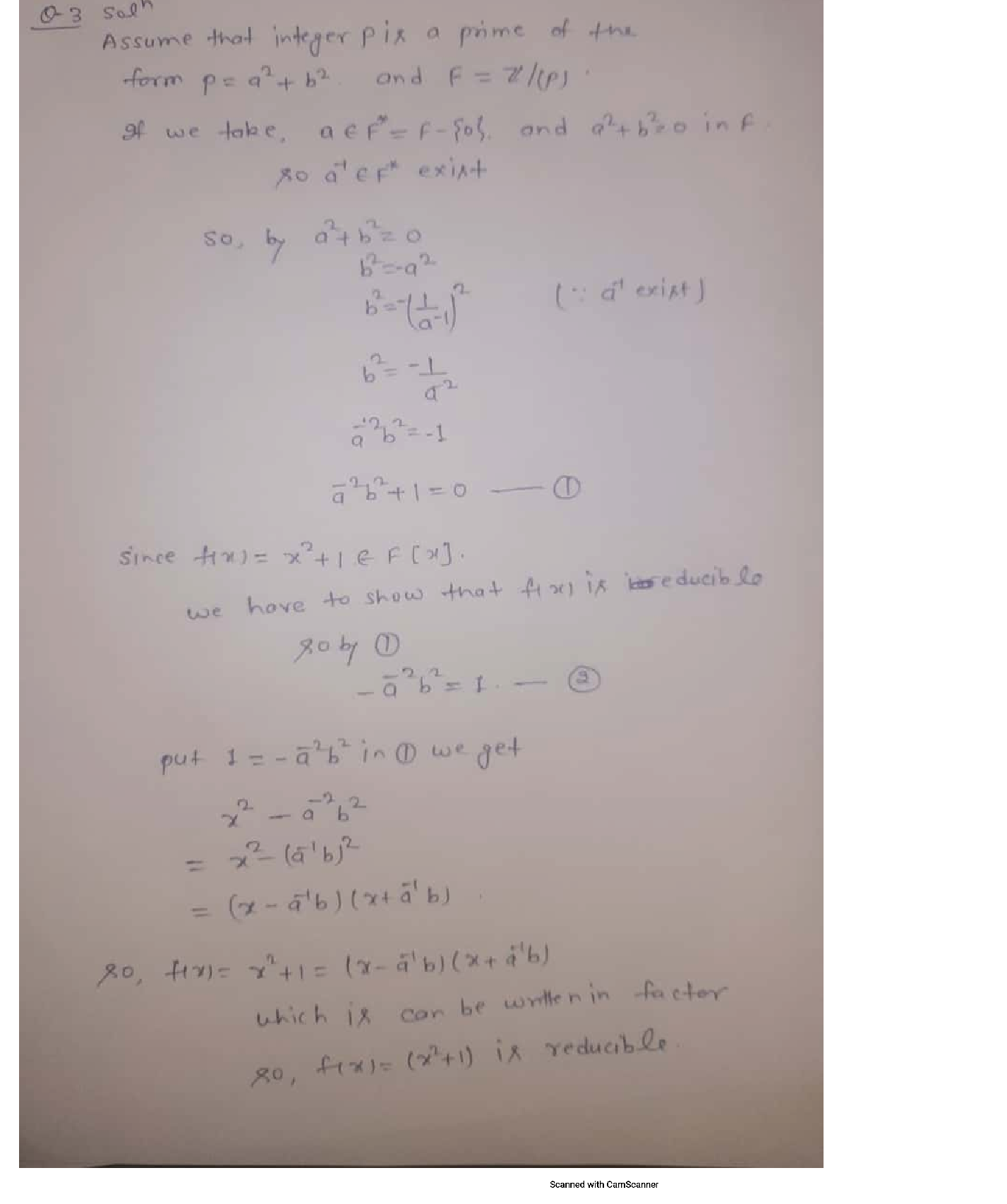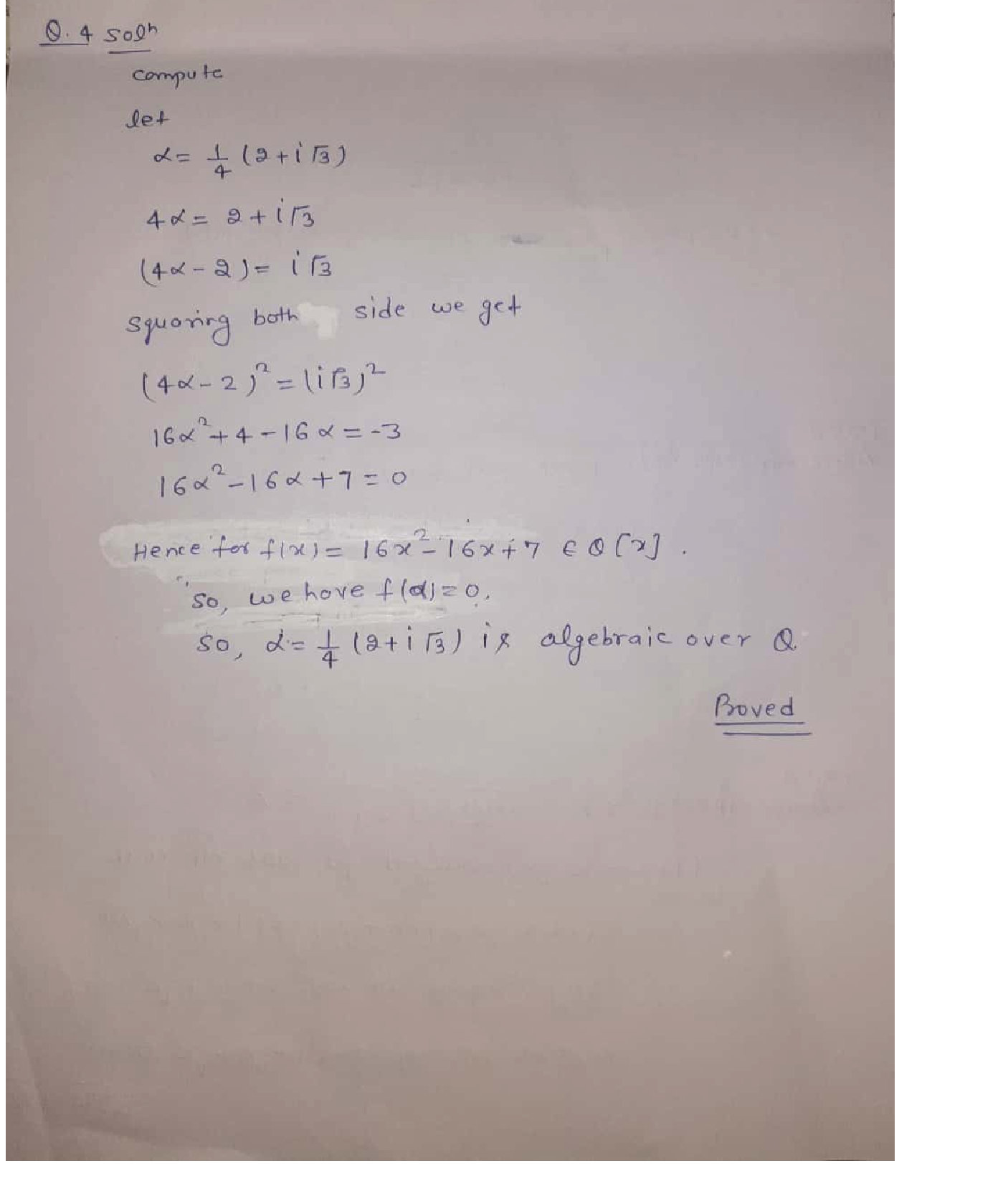### Question 52404Linear Algebra

In an election campaign, the popularity, B, as a percent of voters, of the governing Blue party can be modelled by a function of time, t, in days throughout the campaign as B(t) = 40 - 0.5t. The popularity, R(t), of the opposing Red party can be modelled by a composite function of B(t),R(B(t)) = 20 + 0.75[40 – B(t)].
Graph B(t) and describe the trend.

### Question 45665Linear Algebra

\text { 4] Find } g(0)-g(9)+g(2) \text {, if }
g(x)=\left\{\begin{aligned} \frac{x+1}{2}, & \text { if } x \text { is odd } \\ \frac{x}{2}, & \text { if } x \text { is even } \end{aligned}\right.

### Question 45664Linear Algebra

\text { 3] Find } f(4)-f(2)+f(3) \text {, if }
f(x)=\left\{\begin{array}{ll} \frac{x+1}{2}, & \text { if } x \text { is odd } \\ \frac{x}{4}, & \text { if } x \text { is even } \end{array}\right.

### Question 45663Linear Algebra

2] Find the Domains of the following functions:
\text { a) } f(x)=\sqrt{15-5 x}
f(x)=\frac{x^{2}-2 x+1}{x^{2}-4 x-21}
f(x)=\frac{x^{2}-2 x+1}{\sqrt{16-2 x}}

### Question 45662Linear Algebra

a) Give the definition of a rational function. [5 pts]
b) Give an example of a polynomial function of degree 3. [5 pts]
c) Can a constant function be a polynomial and a rational function at the same time? Explain your answer. [5 pts]
d) Give an example of a non-polynomial function and explain why not apolynomial function. [10 pts)

### Question 45333Linear Algebra

5) Ве,
\begin{array}{c} f: \mathbb{R} \rightarrow \mathbb{R} \\ f(x)=\left\{\begin{array}{ll} x^{2}-3 \cos (\pi x) & x<0 \\ x-4 e^{-2 x} & x \geq 0 . \end{array}\right. \end{array}
Calculate
\int_{-1}^{2} f(x) d x
Presenting the result in simplified form

### Question 45332Linear Algebra

4) Be f: R - Ra function differentiable in R such your derivative f', has in maximum a real zero.
Prove that the equation f(x)=0 has in maximum 2 real square

### Question 45331Linear Algebra

3) Take in consideration the following function
f(x)=\left\{\begin{array}{ll} f: \mathbb{R} \rightarrow \mathbb{R} & \\ \frac{x^{2}-4 x+\cos (\sin (x)),}{x^{4}+4 x^{2}+1}, & x \leq 0 \end{array}\right.
a) Show that the function f is continuous in R+ and in R- but discontinuous in the point X
b) Say justifying, if f is differenciable in X=0

### Question 45330Linear Algebra

2) Prove by definition that
\lim _{x \rightarrow 0} x^{2} \cos \left(e^{x}\right)=0

### Question 45329Linear Algebra

1) Calculate the following limit:
\lim _{x \rightarrow+\infty} \frac{x^{2}\left(e^{-3 x}+1\right)+x \cos (5 x)}{x^{2}+7 x+1}

### Submit query